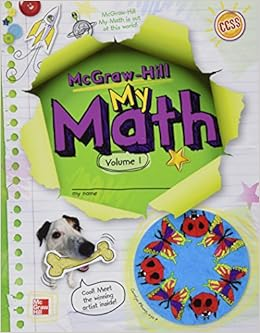My MathClick on pic for some fun practice!

Math Program

Northwest Local Schools has adopted McGraw-Hill’s My Math program. This program was developed to specifically address the State Standards. My Math uses an interactive text where students are engaged in math throughout their learning process (cooperative groups, individual and partner games/activities, dry erase boards, interactive white boards, hands-on activities, classroom computers--just to name a few!).

Our daily math block allows us to explore concepts and skills deeper using a variety of methods! Students will create an interactive Math Notebook to record vocabulary words, as well as other important concepts, which they can refer to during classroom activities.

In fourth grade, students focus on the following mathematical concepts and skills:

Place Value and Money: Numbers up to millions, place value patterns, greater numbers, comparing, and ordering and numbers, size of numbers, using money to understand decimals, counting money and making change, decimals.

Adding and Subtracting Whole Numbers and Money: adding, subtracting using mental math, estimating sums and differences, overestimates and underestimates, adding whole numbers and money, column addition, subtracting whole numbers and money, choose a computation method, translating words to expressions (Algebra), matching words and number expressions (Algebra), evaluating expressions (Algebra), solving addition and subtraction equations (Algebra).

Multiplication and Division Concepts and Facts: meanings for multiplication, patterns in multiplying by 0, 1, 2, 5, and 9, using known facts to find unknown facts, multiplying by 10, 11 and 12, meanings for division, relating multiplication and division, division facts, special quotients, multiplication and division stories, writing and evaluating expressions (Algebra), find a rule (Algebra) and solving multiplication and division equations (Algebra).

Time, Data and Graphs: telling time, units of time, elapsed time, calendars, pictographs, line plots, bar graphs, and line graphs (Algebra).

Multiplying by One-Digit Numbers: multiplying by multiples of 10, 100, or 1,000, estimating products, mental math, using arrays to multiply, multiplying two-digit and one-digit, multiplying three-digit and one-digit numbers, multiplying money, multiplying three factors (Algebra).

Multiplying by Two-Digit Numbers: multiplying multiples of ten, estimating products, using arrays to multiply, multiplying two-digit numbers, multiplying greater numbers, choose a computation method, multiplying money.

Dividing: using patterns to divide mentally, estimating quotients, dividing with remainders, two-digit quotients, dividing two-digit numbers, dividing three-digit numbers, zeros in the quotient, dividing money amounts, divisibility rules, finding averages, dividing by multiples of 10, dividing two-digit divisors.

Geometry and Measurement: relating solids and plane figures, polygons, lines, line segments, rays and angles, triangles and quadrilaterals, circles, congruent figures and motions, symmetry, similar figures, perimeter (Algebra), area (Algebra), volume (Algebra).

Fraction Concepts: parts of a region, parts of a set, fractions, length, and the number line, estimating fractional parts, equivalent fractions, fractions in simplest form, using number sense to compare fractions, comparing and ordering fractions, mixed numbers and improper fractions, comparing mixed numbers, circle graphs.

Fraction Operations and Customary Measurement: estimating fraction sums, adding fractions with like denominators, adding fractions with unlike denominators, subtracting fractions with like denominators, subtracting fractions with unlike denominators, length and customary units, fractions of an inch, capacity and customary units, changing units and comparing measures.

Decimals and Metric Measurement: decimals and fractions, decimal place value, comparing and ordering decimals, rounding decimals, estimating decimal sums and differences, using grids to add and subtract decimals, adding and subtracting decimals, length and metric units, capacity and metric units, mass and metric units, changing units comparing measures.